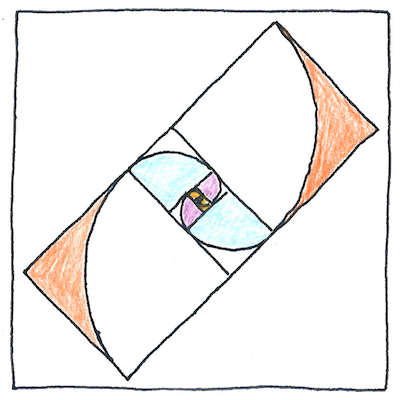# 285 CE Diophantine equation

## The book of science

Tom Sharp

 Diophantus mathematics

## Diophantine equation

• A diophantine equation has more than one unknown.
• We look for only integer solutions.
• We are interested in integers, whole numbers,
• not fractions or continuous lines.
• *
• Diophantine solutions of the Pythagorean theorem
• are Pythagorean triples, (3,4,5), (5,12,13), and so forth.
• Taxicab numbers, starting with 1729, can be expressed
• as the sum of the same number of cubes in two different ways.
• Whatever you multiply one square so the difference
• from another square is 1 are Pell numbers.
• These are the denominators of the closest
• rational approximations to the square root of 2.

• Brahmagupta showed how to solve it in 628.
• Bhāskara II solved it in 1150.
• Narayana Pandit solved it in 1356.
• Pierre de Fermat and William Brouncker solved it in 1657
• but Leonhard Euler named it after John Pell.

## Unknowns

• The number of unknowns
• is greater than the number of equations.
• The number of ways to answer each question
• is greater than the number of questions.
• Wrong ways are more numerous,
• but for many things there are no wrong ways.
• A series of correct answers
• never reaches an imagined convergence.

Pythagorean triples are integer solutions to a2 + b2 = c2 where a < b.

The first taxicab number, 13 + 123 = 93 + 103 = 1729.

Pell numbers are integer solutions to x2 - 2y2 = ±1.